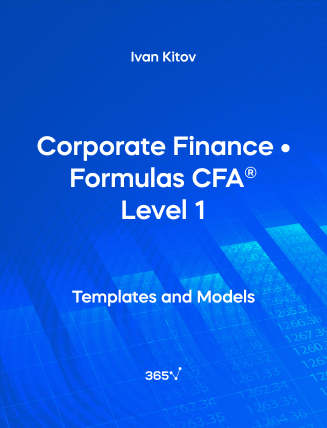## Corporate Finance • Formulas CFA® Level 1###### Ivan Kitov

Need an all-in-one list with the Corporate Finance formulas included in the CFA® Level 1 Exam? We have compiled them for you here. The relevant formulas have been organized and presented by chapter. In this section, we will cover the following topics — Capital Budgeting, Cost of Capital, Measures of Leverage, and Working Capital Management.

## 1. Capital Budgeting

Net present value (NPV)

NPV = \sum_{t=0}^n  \frac {CF{_t}}{(1+r){^t}}

CF{_t} = After-tax cash flow at time t
r = Required rate of return for the investment

Internal Rate of Return (IRR)

\sum_{t=0}^N  \frac {CF{_t}}{(1+IRR){^t}} = 0

Average Accounting Rate of Return (AAR)

AAR = \frac {Average~net~income}{Average~book~value}

Profitability Index (PI)

PI = \frac {PV~of~future~cash~flows}{Initial~Investment} = 1+ \frac {NPV}{Initial~Investment}

## 2. Cost of Capital

Weighted Average Cost of Capital (WACC)

WACC = w{_d}r{_d} (1 - t) + w{_p}r{_p} + w{_e}r{_e}

w{_d} = Proportion of debt that the company uses when it raises new funds
r{_d} = Before-tax marginal cost of debt
t = Company’s marginal tax rate
w{_p} = Proportion of preferred stock the company uses when it raises new funds
r{_p} = The marginal cost of preferred stock
w{_e} = Proportion of equity that the company uses when it raises new funds
r{_e} = Marginal cost of equity

Tax Shield

Tax~shield = Deduction × Tax~rate

Cost of Preferred Stock

r{_p} = \frac {D{_p}}{P{_p}}

P{_p} = Current preferred stock price per share
D{_p} = Preferred stock dividend per share
r{_P} = Cost of preferred stock

Cost of Equity (Dividend discount model approach)

r{_e} = \frac {D{_1}}{P{_0}}+g

P{_0} = Current market value of the equity market index
D{_1} = Dividends expected next period on the index
r{_e} = Required rate of return on the market
g = Expected growth rate of dividends

Growth Rate

g = \bigg( 1- \frac {D}{EPS} \bigg) \times ROE

\frac {D}{EPS} = Assumed stable dividend payout ratio
ROE = Historical return on equity

Cost of Equity (Bond yield plus risk premium)

r{_e} = r{_d} + Risk~Premium

Risk~premium = the additional yield on a company’s stock relative to its bonds

Capital Asset Pricing Model (CAPM)

E (R{_i}) = R{_F} + β{_i} [E (R{_M}) - R{_F}]

β{_i} = The return sensitivity of stock i to changes in the market return
E(R{_M}) = The expected return on the market
E(R{_M}) − R{_F} = The expected market risk premium
R{_F} = Risk-free rate of interest

Beta of a Stock

β{_i} = \frac {Cov (R{_i}, R{_M})}{Var (R{_M})}

R{_M} = Average expected rate of return on the market
R{_i} = Expected return on an asset i
Cov = Covariance
Var = Variance

Pure-play Method Project Beta (De-lever)

β{_{Unlevered(Comparable)}} = \frac {β{_{Levered,~Comparable}}}{\bigg[1+\bigg((1-t{_{Comparable}}) \frac {D{_{Comparable}}}{E{_{Comparable}}}\bigg)\bigg]}

t = Tax rate
D = Debt
E = Equity

Pure-play Method for Subject Firm (Re-lever)

β{_{Levered,~Project}} = {β{_{Unlevered,~Comparable}}}{\bigg[1+\bigg((1-t{_{Project}}) \frac {D{_{Project}}}{E{_{Project}}}\bigg)\bigg]}

E(R{_i}) = R{_F} + β{_i} [E (R{_M}) - R{_F} + Country~risk~premium]

CRP = Sovereign~yield~spread \times \Big(\frac {\sigma~of~equity~index~of~the~developing~country}{\sigma~of~sovereign~bond~market~in~terms~of~the~developed~market~currency}\Big)

σ = Standard deviation

Break Point

Break~point = \frac {Amount~of~capital~at~which~the~source’s~cost~of~capital~changes} {Proportion~of~new~capital~raised~from~the~source}

## 3. Measures of Leverage

Degree of Operating Leverage

Degree~of~Operating~Leverage = \frac {Percentage~change~in~operating~income}{Percentage~change~in~units ~sold}

Degree of Financial Leverage

Degree~of~Financial~Leverage = \frac {Percentage~change~in~Net~Income}{Percentage~change~in~EBIT}

Degree of Total Leverage

Degree~of~Total~Leverage = \frac {Percentage~change~in~Net~Income}{Percentage~change~in~number~of~Units~Sold}

Return on Equity (ROE)

Return~on~Equity = \frac {Net~Income}{Shareholders’~Equity}

The Breakeven Quantity of Sales

Q{_{Breakeven}}= \frac {F + C}{P - V}

P = Price per unit
V = Variable cost per unit
F = Fixed operating costs
C = Fixed financial cost
Q = Quantity of units produced and sold

Operating Breakeven Quantity of Sales

Q{_{Operating~Breakeven}}= \frac {F}{P - V}

P = Price per unit
V = Variable cost per unit
F = Fixed operating costs

## 4. Working Capital Management

Current Ratio

Current~Ratio = \frac {Current~assets}{Current~liabilities}

Quick Ratio

Quick~Ratio = \frac {Cash + Receivables + Short–term~marketable~investments}{Current~liabilities}

Accounts Receivable Turnover

Accounts~Receivable~Turnover= \frac {Credit~sales}{Average~receivables}

Number of Days of Receivables

Number~of~days~of~receivables = \frac {365}{Accounts~receivable~turnover}

Inventory Turnover

Inventory~Turnover = \frac {Cost~of~goods~sold}{Average~Inventory}

Number of Days of Inventory

Number~of~days~of~Inventory = \frac {365}{Inventory~turnover}

Payables Turnover

Payables~Turnover~Ratio = \frac {Purchases}{Average~accounts~payables}

Number of Days of Payables

Number~of~days~of~Payables = \frac {365}{Payables~turnover~ratio}

Net Operating Cycle

Net~operating~cycle = Number~of~days~of~inventory+ Number~of~days~of~receivables - Number~of~days~of~payables

Yield on a Bank Discount Basis (BDY)

r{_{BD}} = \frac {D}{F} \times \frac {360}{t}

D = Dollar discount, which is equal to the difference between the face value of the bill (F) and its purchase price (P0)
F = Face value of the T-bill
t = Actual number of days remaining to maturity
r{_{BD}} = Annualized yield on a bank discount basis

Effective Annual Yield (EAY)

EAY = ( 1 + HPR){^{\frac {360}{t}}} - 1

Holding Period Return

HPR = \frac {(Cashflow~ending~value - Beginning~value + Cashflow~received)}{Beginning~value}

Cost~of trade~credit = \Bigg( 1+\frac {\%Discount}{1 - \%Discount} \Bigg){^{\frac {360}{Number~of~days~past~discount}}} - 1
Cost~of~borrowing = \frac {Interest + Dealer’s~commission + Other~costs}{Loan~amount - Interest}